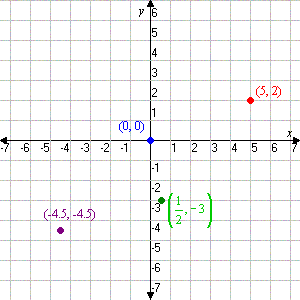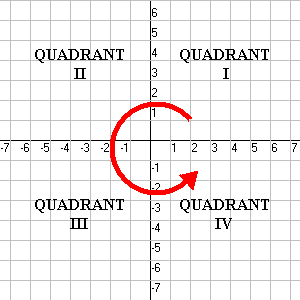# Plotting Points on a Cartesian Plane

A Cartesian plane (named after French mathematician Rene Descartes, who formalized its use in mathematics) is defined by two perpendicular number lines: the-axis, which is horizontal, and the-axis, which is vertical. Using these axes, we can describe any point in the plane using an ordered pair of numbers.

The Cartesian plane extends infinitely in all directions. To show this, math textbooks usually put arrows at the ends of the axes in their drawings.

The location of a point in the plane is given by its coordinates, a pair of numbers enclosed in parentheses:. The first numbergives the point's horizontal position and the second numbergives its vertical position. All positions are measured relative to a "central" point called the origin, whose coordinates are. For example, the pointisunits to the right of the origin andunits up, as shown in the figure. Negative coordinate numbers tell us to go left or down. See the other points in the figure for examples.The Cartesian plane is divided into four quadrants. These are numbered fromthrough, starting with the upper right and going around counterclockwise. (For some reason everybody uses roman numerals for this).In Quadrant, both the- and-coordinates are positive; in Quadrant, the-coordinate is negative, but the-coordinate is positive; in Quadrantboth are negative; and in Quadrant IVis positive butis negative.

Points which lie on an axis (i.e., which have at least one coordinate equal to) are said not to be in any quadrant. Coordinates of the formlie on the horizontal-axis, and coordinates of the formlie on the vertical-axis.